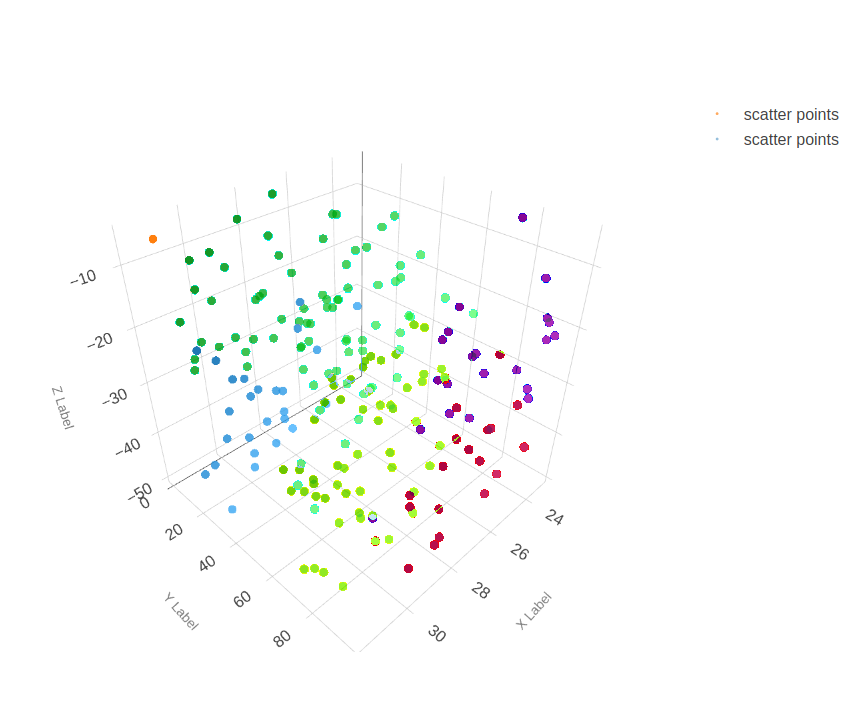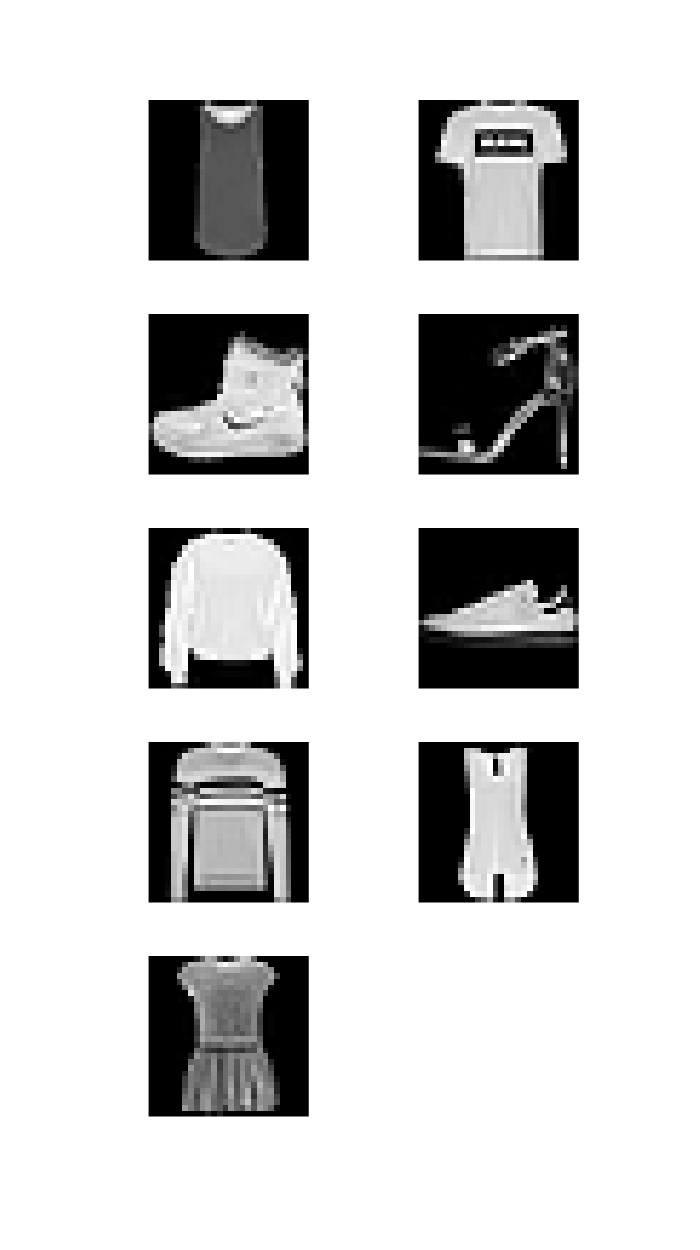# Operation Feedback¶

Operations can provide a variety of forms of real-time feedback including subplots, 2D and 3D plots and images using `matplotlib`.

## Graphs¶

The following example shows a sample 3D scatter plot and its rendering in DeepForge.

```import matplotlib.pyplot as plt
import numpy as np
from mpl_toolkits.mplot3d import Axes3D

class Scatter3DPlots():

def execute(self):
# Set random seed for reproducibility
np.random.seed(19680801)

def randrange(n, vmin, vmax):
'''
Helper function to make an array of random numbers having shape (n, )
with each number distributed Uniform(vmin, vmax).
'''
return (vmax - vmin)*np.random.rand(n) + vmin

fig = plt.figure()
ax = fig.add_subplot(111, projection='3d')

n = 100

# For each set of style and range settings, plot n random points in the box
# defined by x in [23, 32], y in [0, 100], z in [zlow, zhigh].
for m, zlow, zhigh in [('o', -50, -25), ('^', -30, -5)]:
xs = randrange(n, 23, 32)
ys = randrange(n, 0, 100)
zs = randrange(n, zlow, zhigh)
ax.scatter(xs, ys, zs, marker=m)

ax.set_xlabel('X Label')
ax.set_ylabel('Y Label')
ax.set_zlabel('Z Label')
plt.show()
```Example of a 3D scatter plot using matplotlib in DeepForge

## Images¶

Visualizing images using matplotlib is also supported. The following example shows images from the MNIST fashion dataset.

```from matplotlib import pyplot
from keras.datasets import fashion_mnist

class MnistFashion():

def execute(self):

(trainX, trainy), (testX, testy) = fashion_mnist.load_data()
# summarize loaded dataset
print('Train: X=%s, y=%s' % (trainX.shape, trainy.shape))
print('Test: X=%s, y=%s' % (testX.shape, testy.shape))
for i in range(9):
pyplot.subplot(330 + 1 + i)  # define subplot
pyplot.imshow(trainX[i], cmap=pyplot.get_cmap('gray'))  # plot raw pixel data
pyplot.show()
```Visualizing MNIST fashion images in DeepForge